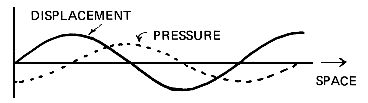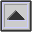SOUND PRESSURE

The atmosphere exerts pressure on all objects in it. When a vibrating body moves in air, it creates slight disturbances of the AMBIENT atmospheric pressure. The AMPLITUDE of these pressure variations (that is, their maximum displacement from the ambient atmospheric pressure) is called the sound pressure variation, whereas the effective pressure variation is 0.707 the maximum value (see ROOT MEAN SQUARE). The oscillating variations in sound pressure (called the WAVEFORM of the sound) PROPAGATE in the form of a SOUND WAVE.

When the amplitude of the vibrating body, such as a tuning fork, is greatest, its velocity is zero (that is, it has reached its outer limit of displacement and is momentarily motionless before returning in the opposite direction). If the velocity is zero, so is the pressure it exerts on the medium (e.g. air) around it. Velocity (and therefore pressure) is greatest mid-way between the maximum displacement of the vibrating body, and we can graph the resulting relationship between amplitude and sound pressure in the following way:Sound pressure variation of a sine wave showing the PHASE relationship between pressure and particle displacement.

For other versions of this graph, see CYCLE and SIMPLE HARMONIC MOTION.

It is the sound pressure rather than the actual physical INTENSITY of the wave which our EARDRUMs and MICROPHONEs react to. SOUND INTENSITY is proportional to the square of the sound pressure and so we may calculate intensity (which is difficult to measure) by measuring sound pressure (which is relatively simple to measure).

See: DECIBEL, SOUND LEVEL, SOUND PRESSURE LEVEL, THRESHOLD OF HEARING, TRANSDUCER. Compare: LOUDNESS, POWER, VOLUME.

Sound pressure may be measured in dynes per square centimeter (dynes/cm2) or Newtons per square meter (N/m2) where 1 N/m2 = 10 dynes/cm2 @ 10-5 atmospheric pressure. See Appendix D for the conversion of pressure ratios to decibels.

home